Related Articles
Class 8 RD Sharma Solutions – Chapter 3 Squares and Square Roots – Exercise 3.7 | Set 1
• Last Updated : 06 Apr, 2021

### Question 1) 84.8241

Solution: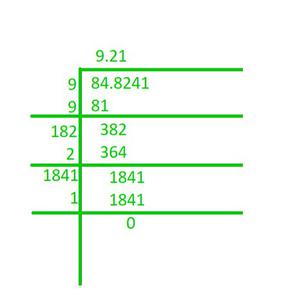Hence, the square root of 84.8241 is 9.21.

### Question 2) 0.7225

Solution: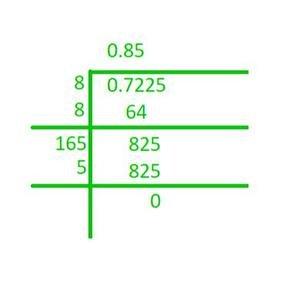Hence, the square root of 0.7225 is 0.85.

### Question 3) 0.813604

Solution: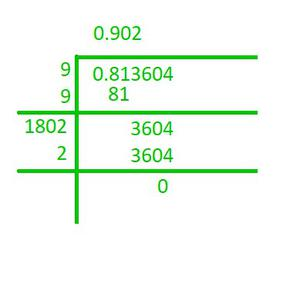Hence, the square root of 0.813604 is 0.902.

### Question 4) 0.00002025

Solution: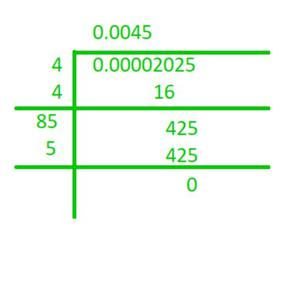Hence, the square root of 0.00002025 is 0.0045.

### Question 5) 150.0625

Solution: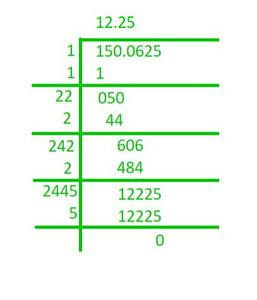Hence, the square root of 150.0625 is 12.25.

### Question 6) 225.6004

Solution: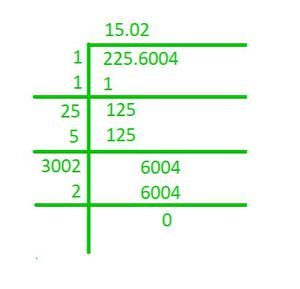Hence, the square root of 225.6004 is 15.02.

### Question 7) 3600.720036

Solution: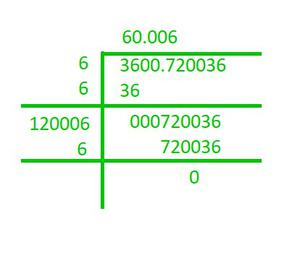Hence, the square root of 3600.720036 is 60.006.

### Question 8) 236.144689

Solution: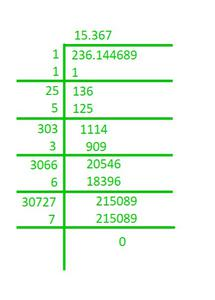Hence, the square root of 236.144689 is 15.367.

### Question 9) 0.00059049

Solution: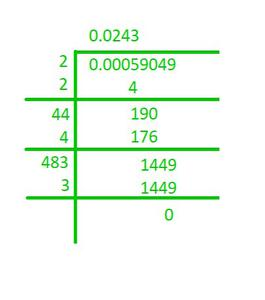Hence, the square root of 0.00059049 is 0.0243.

### Question 10) 176.252176

Solution:Hence, the square root of 176.252176 is 13.276.

### Chapter 3 Squares and Square Roots – Exercise 3.7 | Set  2

My Personal Notes arrow_drop_up